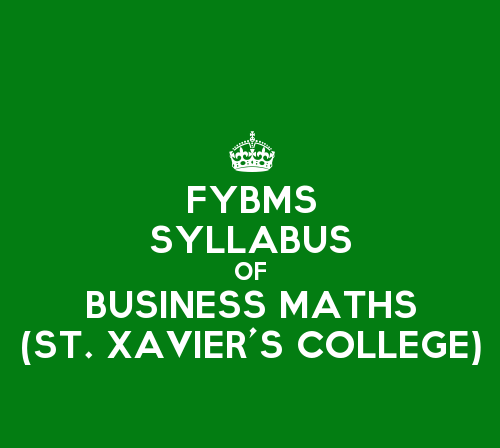# St. Xavier’s College FYBMS Sem 1 Syllabus: Business Mathematics

0

Business Mathematics is a subject of Semester 1 in FYBMS at St. Xavier’s College. Business Mathematics subject aims to equip the BMS students with a broad based knowledge of mathematics with emphasis on business applications.

There are 4 main units in the subject of Business Mathematics:

Unit 1 – Elementary Financial Mathematics – 15 lectures

Unit 2 – Matrices and Determinants – 15 lectures

Unit 3 – Derivatives and their Applications – 15 lectures

Unit 4 – Functions of two variables and Integration – 15 lectures

The topics covered in Unit 1 – Elementary Financial Mathematics are as follows:

1. A.P., G.P., and sums of their first n terms.
3. Simple interest, compound interest, interest compounded more than once a year nominal,
4. Effective and continuous rates of interest.
5. Immediate (ordinary) annuity, its present value and future value.
6. Equated Monthly installments (EMI) using reducing interest system,
7. Amortization of loans.
8. Sinking fund.
9. Depreciation of assets.
10. Functions: constant function, linear function, step function, xn, exponential and logarithmic functions.
11. Business and Economics functions such as demand supply, total revenue, average revenue, total cost, average cost and profit function.
12. Equilibrium point.
13. Break even analysis.
14. Notion of permutations and combinations.
15. (Problems to be solved with calculator. Use of logarithm tables to be avoided)

The topics covered in Unit 2 – Matrices and Determinants are as follows:

1. Matrices,
2. Matrix Operations (Addition of 2 matrices, scalar multiple of a matrix, matrix multiplication, transpose of a matrix).
3. Determinant of a matrix of order 2 and 3,
4. Elementary properties of determinant,
5. Solving a system of linear equations (upto 3 variable) using Cramer’s rule and application to business problems.
6. Elementary row and column operations on matrices,
7. Inverse of a matrix (up to order 3),
8. Application of matrices to Leontief’s open input-output model,
9. Linear inequality (upto 2 variables) and their graphs.

The topics covered in Unit 3 – Derivatives and their Applications are as follows:

1. Derivatives as a measure of rate.
2. Derivatives of functions – constant function,
3. X”, ex, ax, log x.
4. Rules of derivatives – Scalar multiplication, sum, difference, product and quotient.
5. Second order derivatives.
6. Application of derivatives – marginal cost, marginal revenue, elasticity of demand, maxima and minima for functions in Economics and Business.

The topics covered in Unit 4 – Functions of two variables and Integration are as follows:

1. Functions of two variables with examples from Economics and Commerce such as Cobb-Douglas and other production functions,
2. Demand functions involving 2 commodities.
3. First and second order partial derivatives,
4. Marginal functions and their simple applications in Economics.
5. Maxima and Minima of functions of two variable using second order partial derivative criterion.
6. Constrained maxima and minima (one constraint only) using Lagrange’s multiplier.
7. Simple applications in Economic and Commerce:
8. Marginal physical productivity of labour and capital,
9. Demand analysis of complementary and competitive commodities,
10. Partial elasticity,
11. Optimization of functions of two variables in economics and business.
12. Integration as the reverse process of derivatives,
13. Standard formulae – integration of constant function, xn, ex, ax, log x.
14. Rules for integrals – sum, difference and scalar multiplications.
15. Simple problems (Integration techniques such as finding total cost from marginal cost, total revenue from marginal revenue)
16. Definite integrations, simple problems (no properties),
17. Applications such producer’s surplus,
18. Consumer’s surplus,
19. Present value and future value of a continuously compounded annuity.

Continuous Internal Assessment:

1. Mid Semester Test
2. Assignment

The list of Reference Books for Business Mathematics are:

1. Mathematics for Economics and Finance, Martin Anthony, Norman Biggs Cambridge low-price editions, 2000.
2. Business Mathematics, D.C. Sancheti, V.K. Kapoor, Sultan Chand & Sons Publications, 2006.
3. Mathematics for Business and Economics, J.D. Gupta, P.K. Gupta, Man Mohan Tata McGraw-Hill Publishing company Ltd.
4. Mathematical Basis of Life Assurance IC – 81: Insurance Institute of India, 2006.
5. Mathematics of Finance 2nd Edition Schaum’s Outline Series, Peter Zima, Robert Brows Tata McGraw – Hill Publishing Co. Ltd.## Like it? Share with your friends!

0

Choose A Format
Personality quiz
Series of questions that intends to reveal something about the personality
Trivia quiz
Series of questions with right and wrong answers that intends to check knowledge
Poll
Voting to make decisions or determine opinions
Story
Formatted Text with Embeds and Visuals
List
The Classic Internet Listicles
Countdown
The Classic Internet Countdowns
Open List
Submit your own item and vote up for the best submission
Ranked List
Upvote or downvote to decide the best list item
Meme